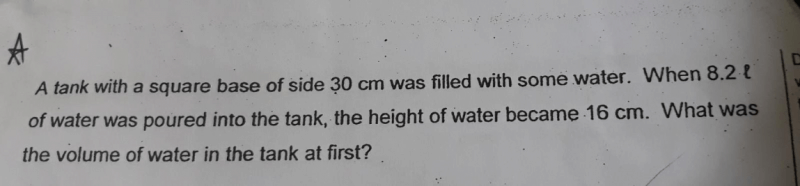QuestionCan you anyone help to explain the solution of this sum?

Volume = Base Area x Height

Since the base area is a square,
Base Area = 30 cm x 30 cm
= 900 cm2.

So to find the volume when the height is 16cm:
Volume = 900 cm2 x 16 cm
= 14,400 cm3 or 14,400 ml

Volume of water at first = 14,400 ml – 8,200 ml
= 6,200 ml or 6.2 l